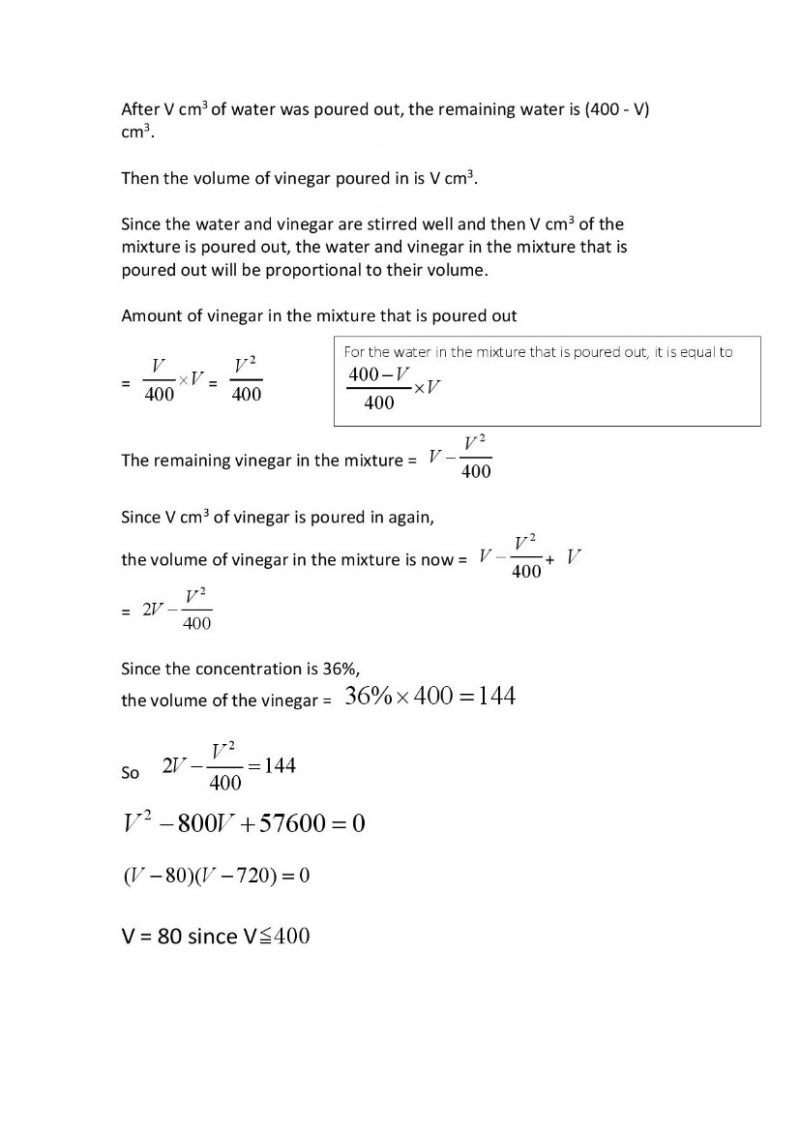# Question

Hi, need help for the following question on quadratic equation:

A beaker has 400 cubic centimetres of water. V cubic centimetres of water is poured out and then replaced by the same amount of vinegar.  After stirring well, V cubic centimetres of the first mixture is poured out and replaced by the same amount of vinegar again. The percentage of vinegar in the final mixture is 36%. Find the value of V.

Thanks.

Source: ?

4 Answers

# Answer0 Replies 2 Likes ✔Accepted Answer
Amount of vinegar in the first mixture = V/400
(V/400) x V = (V^2)/400 (amount of vinegar in the first mixture that is poured out)
V – [(V^2)/400] (amount of vinegar remaining in the first mixture)
V – [(V^2)/400] + V = 2V –  [(V^2)/400] (amount of vinegar in the final mixture)
2V –  [(V^2)/400] = 56% x 400 = 144
800V – V^2 = 57600
V^2 – 800V + 57600 = 0
(V – 720)(V – 80) = 0
V = 720 (reject as V is less than 400)
V = 80 (accept)

Ans : 80 cm^3.

0 Replies 0 Likes

Hi THW, could I have the detailed working please? Thanks.

1 Reply 0 Likes

Please accept the solution.

Thank you.

0 Replies 0 Likes

V = 80

0 Replies 0 Likes